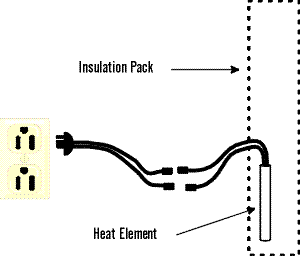Hot wiring refers to simply connecting the 120 volt heat element straight to 120 volt power. Hot wiring by-passes all other controls and eliminates them as possible problems when testing the cooling unit.

To hot wire, disconnect the electrical connections between the refrigerator and the 120 volt heat element (leaving the heat element in place inside the insulation pack of the cooling unit) and then by whatever safe means necessary connect the heat element straight to 120 volt power. If the heat element gets hot (you can tell by lightly touching the insulation pack after about a half hour of operation) you can be better than 95% sure that you have met the correct heat requirement of the three requirements for a cooling unit to operate. If the cooling unit fails to operate, it will be necessary to be absolutely sure that the heat element is producing the right heat. Each model of refrigerator requires a certain wattage heat element to operate. This wattage is critical for operation (within 5% on some models). You will need to determine the correct wattage for the refrigerator and then test for it using one of the following instruments: watt meter, amp meter, or ohm meter. All of the necessary numbers can be found elsewhere in this program by model number. The watt meter and amp meter are used when the heat element is connected to power. The ohm meter is used when the heat element is not connected to power. It will be necessary to be familiar with the instrument you are using.

Hot wiring is pretty straight forward if you have only one heat element (120 volt) with only two wires. You connect the two wires to the 120 volt power, and it doesn't matter which wire goes where (no polarity). However, not all refrigerators are that simple. Below are other possible configurations, along with a few clues that might help locate the 120 volt wires necessary for hot wiring. In some cases it may be necessary to locate a wiring diagram.

If your refrigerator has two heat elements, one is 120 volt and the other is 12 volt. The heat element with the smaller gauge wire (if there is a difference) is the 120 volt element. Also, one wire on the 12 volt (low voltage) element may be connected to the side of the heat element (ground) instead of going to the inside of the element.

A three wire heat element presents two possibilities. Either it is a combination 120 volt / 12 volt element, or it is a two stage 120 volt only element. Both styles use three wires, but are wired differently. The simplest way to determine which element you have is to know the capabilities of your refrigerator. If 120 volt is the only electric option you have, then the element would be a two stage 120 volt element. If the refrigerator is capable of both 120 volt and 12 volt operation, the element is a combination element. If the combination element has a red wire, that wire is 120 volt. If it has a yellow wire, that wire is 12 volt. The third wire would be the "common" wire. To hot wire this combination element, you use the red and "common" wire for the connection to the 120 volt power. If these color codes don't correspond to your element, then you will need an ohm meter or wiring diagram.

A two stage heat element (three wire) has a common wire and two "hot" wires. One "hot" wire is for low heat and the other is for high heat. For the cooling unit to work both the low and high heats have to be functioning. In hot wiring this element, the two "hot" wires are tied together as one wire of the connection and the common wire is used for the other connection. To know which wires are which you will need a wire diagram or ohm meter. Also, this type of element can easily mislead you when testing the cooling unit, since even if only one stage of the element were working, the cooling unit insulation pack would still get warm. This, of course, would not be the CORRECT heat necessary to make the cooling unit operate. An amp meter clamped around the common wire will tell you if your pulling the right amperage.

A four wire heat element is a 120 volt / 12 volt element with two separate circuits for each side. If two wires are the same color and the other two wires are each a unique color, then the two wires that are the same color are the 120 volt side of the element. Otherwise, you will be forced to use an ohm meter or locate a wire diagram to determine the right wires.